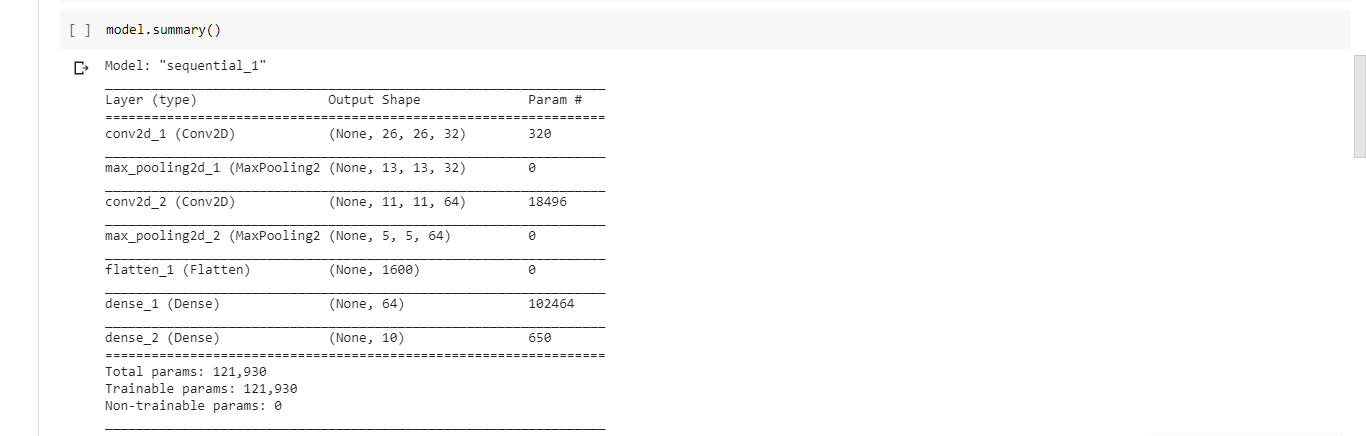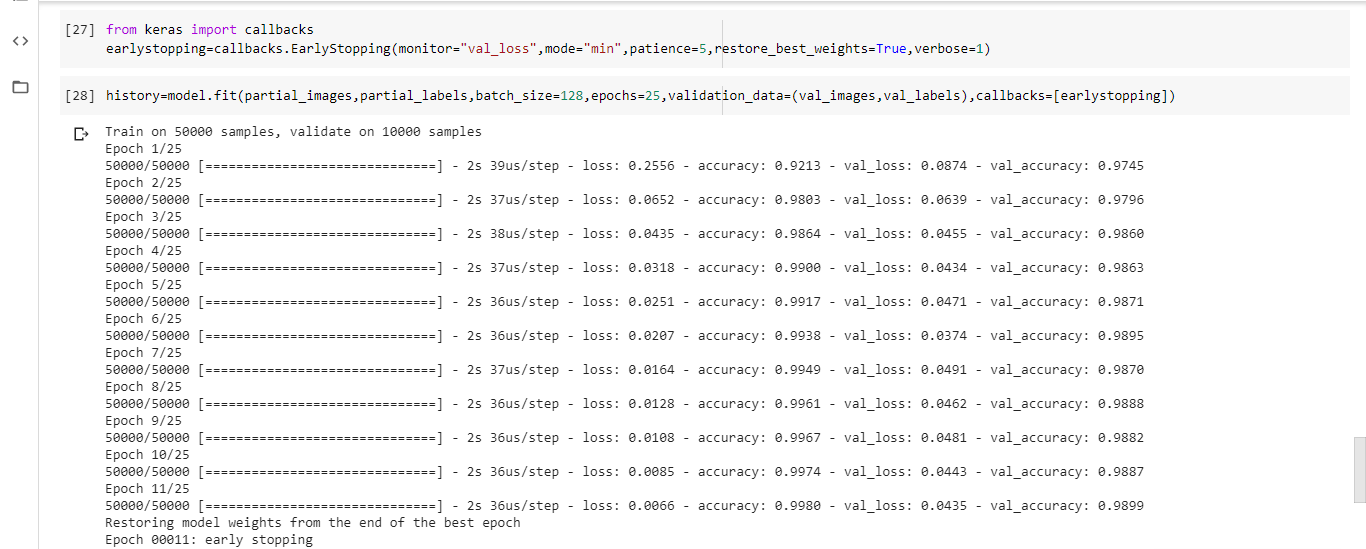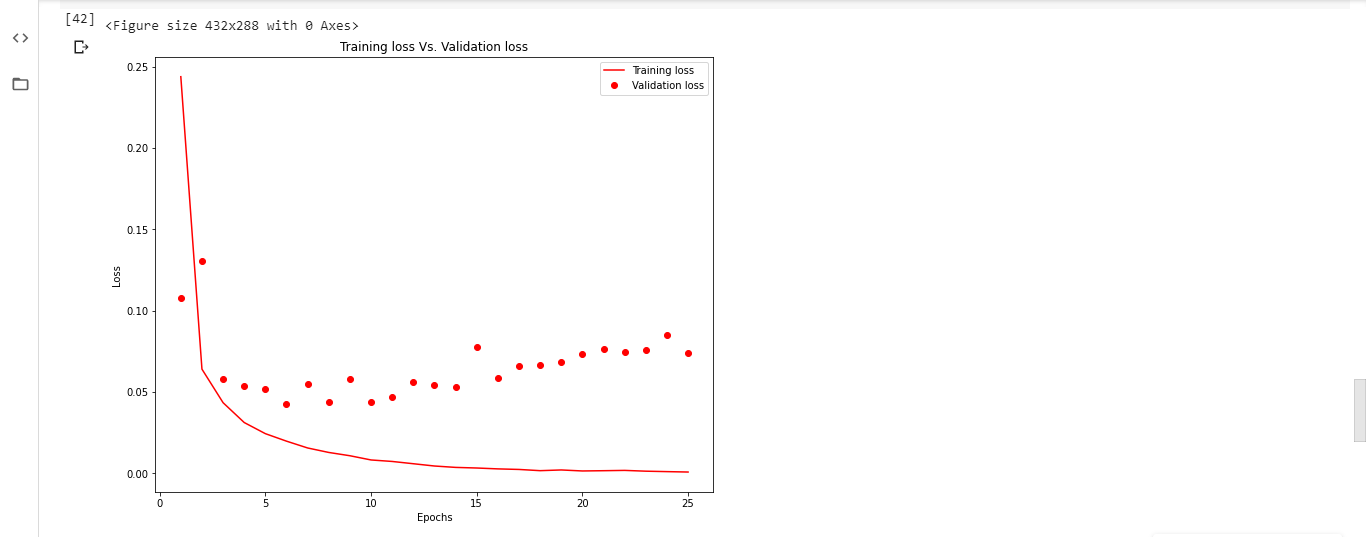# Choose optimal number of epochs to train a neural network in Keras

One of the critical issues while training a neural network on the sample data is Overfitting. When the number of epochs used to train a neural network model is more than necessary, the training model learns patterns that are specific to sample data to a great extent. This makes the model incapable to perform well on a new dataset. This model gives high accuracy on the training set (sample data) but fails to achieve good accuracy on the test set. In other words, the model loses generalization capacity by overfitting to the training data.

To mitigate overfitting and to increase the generalization capacity of the neural network, the model should be trained for an optimal number of epochs. A part of training data is dedicated for validation of the model, to check the performance of the model after each epoch of training. Loss and accuracy on the training set as well as on validation set are monitored to look over the epoch number after which the model starts overfitting.

## keras.callbacks.callbacks.EarlyStopping()

Either loss/accuracy values can be monitored by Early stopping call back function. If the loss is being monitored, training comes to halt when there is an increment observed in loss values. Or, If accuracy is being monitored, training comes to halt when there is decrement observed in accuracy values.

Syntax with default values:

keras.callbacks.callbacks.EarlyStopping(monitor=’val_loss’, min_delta=0, patience=0, verbose=0, mode=’auto’, baseline=None, restore_best_weights=False)

Understanding few important arguments:

• monitor: The value to be monitored by the function should be assigned. It can be validation loss or validation accuracy.
• mode: It is the mode in which change in the quantity monitored should be observed. This can be ‘min’ or ‘max’ or ‘auto’. When the monitored value is loss, its value is ‘min’. When the monitored value is accuracy, its value is ‘max’. When the mode is set is ‘auto’, the function automatically monitors with the suitable mode.
• min_delta: The minimum value should be set for the change to be considered i.e., Change in the value being monitored should be higher than ‘min_delta’ value.
• patience: Patience is the number of epochs for the training to be continued after the first halt. The model waits for patience number of epochs for any improvement in the model.
• verbose: Verbose is an integer value-0, 1 or 2. This value is to select the way in which the progress is displayed while training.
• Verbose = 0: Silent mode-Nothing is displayed in this mode.
• Verbose = 1: A bar depicting the progress of training is displayed.
• Verbose = 2: In this mode, one line per epoch, showing the progress of training per epoch is displayed.
• restore_best_weights: This is a boolean value. True value restores the weights which are optimal.

Finding the optimal number of epochs to avoid overfitting on MNIST dataset.

 `import` `keras ` `from` `keras.utils.np_utils ``import` `to_categorical ` `from` `keras.datasets ``import` `mnist ` ` `  `# Loading data ` `(train_images, train_labels), (test_images, test_labels)``=` `mnist.load_data() ` ` `  `# Reshaping data-Adding number of channels as 1 (Grayscale images) ` `train_images ``=` `train_images.reshape((train_images.shape[``0``],  ` `                                     ``train_images.shape[``1``],  ` `                                     ``train_images.shape[``2``], ``1``)) ` ` `  `test_images ``=` `test_images.reshape((test_images.shape[``0``],  ` `                                   ``test_images.shape[``1``], ` `                                   ``test_images.shape[``2``], ``1``)) ` ` `  `# Scaling down pixel values ` `train_images ``=` `train_images.astype(``'float32'``)``/``255` `test_images ``=` `test_images.astype(``'float32'``)``/``255` ` `  `# Encoding labels to a binary class matrix ` `y_train ``=` `to_categorical(train_labels) ` `y_test ``=` `to_categorical(test_labels) `

Step 2: Building a CNN model

 `from` `keras ``import` `models ` `from` `keras ``import` `layers ` ` `  `model ``=` `models.Sequential() ` `model.add(layers.Conv2D(``32``, (``3``, ``3``), activation ``=``"relu"``,  ` `                             ``input_shape ``=``(``28``, ``28``, ``1``))) ` `model.add(layers.MaxPooling2D(``2``, ``2``)) ` `model.add(layers.Conv2D(``64``, (``3``, ``3``), activation ``=``"relu"``)) ` `model.add(layers.MaxPooling2D(``2``, ``2``)) ` `model.add(layers.Flatten()) ` `model.add(layers.Dense(``64``, activation ``=``"relu"``)) ` `model.add(layers.Dense(``10``, activation ``=``"softmax"``)) ` ` `  `model.summary() `

Output: Summary of the modelStep 4: Compiling the model with RMSprop optimizer, categorical cross entropy loss function and accuracy as success metric

 `model.``compile``(optimizer ``=``"rmsprop"``, loss ``=``"categorical_crossentropy"``, ` `                                             ``metrics ``=``[``'accuracy'``]) `

Step 5: Creating validation set and training set by partitioning the current training set

 `val_images ``=` `train_images[:``10000``] ` `partial_images ``=` `train_images[``10000``:] ` `val_labels ``=` `y_train[:``10000``] ` `partial_labels ``=` `y_train[``10000``:] `

Step 6: Initializing earlystopping callback and training the model

 `from` `keras ``import` `callbacks ` `earlystopping ``=` `callbacks.EarlyStopping(monitor ``=``"val_loss"``,  ` `                                        ``mode ``=``"min"``, patience ``=` `5``,  ` `                                        ``restore_best_weights ``=` `True``) ` ` `  `history ``=` `model.fit(partial_images, partial_labels, batch_size ``=` `128``,  ` `                    ``epochs ``=` `25``, validation_data ``=``(val_images, val_labels),  ` `                    ``callbacks ``=``[earlystopping]) `Training stopped at 11th epoch i.e., the model will start overfitting from 12th epoch. Therefore, the optimal number of epochs to train most dataset is 11.

Observing loss values without using Early Stopping call back function:
Train the model up until 25 epochs and plot the training loss values and validation loss values against number of epochs. The plot looks like:Inference:
As the number of epochs increases beyond 11, training set loss decreases and becomes nearly zero. Whereas, validation loss increases depicting the overfitting of the model on training data.

References:

• https://keras.io/callbacks/
• https://keras.io/datasets/

My Personal Notes arrow_drop_upCheck out this Author's contributed articles.

If you like GeeksforGeeks and would like to contribute, you can also write an article using contribute.geeksforgeeks.org or mail your article to contribute@geeksforgeeks.org. See your article appearing on the GeeksforGeeks main page and help other Geeks.

Please Improve this article if you find anything incorrect by clicking on the "Improve Article" button below.

Article Tags :
Practice Tags :

2

Please write to us at contribute@geeksforgeeks.org to report any issue with the above content.